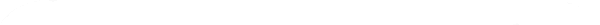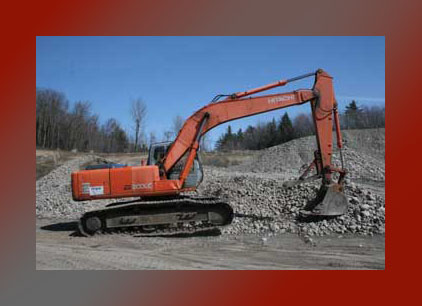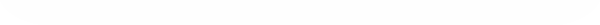Quality aggregates at affordable prices! Home Products Location & Payment Hours Contact Facts Facts and Figures 1 metric ton equals about 2204 pounds 1 cubic foot of sand or gravel weighs approx. 100 lb. (45 kg.) wet or dry A cubic foot of 5/8" crushed gravel weighs approx. 95 lb. (43 kg.) wet or dry A cubic yard of sand or gravel weighs approx. 2700 lb. (1215 kg) wet or dry A cubic yard of 5/8" crushed gravel weighs approx. 2600 lb. (1170 kg) wet or dry A cubic yard has a volume of 27 cubic feet. (.76 Cubic Meters) Area of a circle = Radius (one half of the circle diameter) squared (radius times itself) times (3.1416) expressed in decimal figures. Example: The area of circle with a diameter of 15'6" is, the radius - One half of 15'6" = (7'9") 7.75 x 7.75=60.06 x 3.1416=188.68 sq. ft. Volume of a cylinder = Cylinder's area, determined by the method above, times it's height. Example: Volume of a 16" diameter cylinder, 8'4" high is (16") 1/4 x .5 = (8") .67 x .67 = .45 x 3.1416 = 1.41 x (8'4") 8.33=11.75 cu. ft.Webdesign Copyright :Natalie Gilbert Last Updated: May 2010